Olympiad Test: Cubes and Dice

# Olympiad Test: Cubes and Dice

Test Description

## 15 Questions MCQ Test Science Olympiad Class 9 | Olympiad Test: Cubes and Dice

Olympiad Test: Cubes and Dice for Class 9 2022 is part of Science Olympiad Class 9 preparation. The Olympiad Test: Cubes and Dice questions and answers have been prepared according to the Class 9 exam syllabus.The Olympiad Test: Cubes and Dice MCQs are made for Class 9 2022 Exam. Find important definitions, questions, notes, meanings, examples, exercises, MCQs and online tests for Olympiad Test: Cubes and Dice below.
Solutions of Olympiad Test: Cubes and Dice questions in English are available as part of our Science Olympiad Class 9 for Class 9 & Olympiad Test: Cubes and Dice solutions in Hindi for Science Olympiad Class 9 course. Download more important topics, notes, lectures and mock test series for Class 9 Exam by signing up for free. Attempt Olympiad Test: Cubes and Dice | 15 questions in 15 minutes | Mock test for Class 9 preparation | Free important questions MCQ to study Science Olympiad Class 9 for Class 9 Exam | Download free PDF with solutions
 1 Crore+ students have signed up on EduRev. Have you?
Olympiad Test: Cubes and Dice - Question 1

### A cube painted blue on all faces is cut into 27 small cubes of equal size. How many small cubes are painted on one face only?

Detailed Solution for Olympiad Test: Cubes and Dice - Question 1

The big cube can be cut into 27 small cubes as shown in the figure.
Number of cubes only one face painted = (m − 2)2 × 6
= (3 − 2)2 × 6 = (1)2 × 6 = 6.

Olympiad Test: Cubes and Dice - Question 2

### A cube is painted red on all faces is cut into 27 small cubes of equal size then how many cubes are not painted on any face?

Detailed Solution for Olympiad Test: Cubes and Dice - Question 2

There is only one cube which is not painted on any face.

Olympiad Test: Cubes and Dice - Question 3

### A cube of side 10cm is coloured green with a 2 cm wide red strip along all the sides on all the faces. The cube is cut into 125 smaller cubes of equal size then how many cubes are without any colour?

Detailed Solution for Olympiad Test: Cubes and Dice - Question 3

There are 9 central cubes in three middle layers, which are without any colour.
∴ No. of cubes without any colour = 9 × 3 = 27

Olympiad Test: Cubes and Dice - Question 4

How many dots are there on the dice face opposite the one with three dots?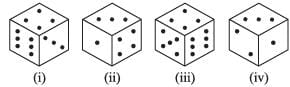Detailed Solution for Olympiad Test: Cubes and Dice - Question 4

From figures (i), (ii), (iv) we observe that 6, 4, 3, 1 lie adjacent to 2. Hence the number 5 will appear on the face showing 2.

Olympiad Test: Cubes and Dice - Question 5

Three positions of a dice are given. Based on them find out which number is found opposite the number 2 in the given cube.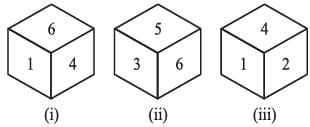Detailed Solution for Olympiad Test: Cubes and Dice - Question 5

From figures (i) & (ii), it is clear that 1, 4, 3, 5 lie adjacent to number 6. So, number 2 lies opposite to 6.

Olympiad Test: Cubes and Dice - Question 6

In the given figure find the number of dots on the face opposite the face having 3 dots.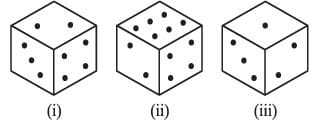Detailed Solution for Olympiad Test: Cubes and Dice - Question 6

From figures (i), (ii) and (iii), we conclude that 3, 4, 6 and 1 dots appear adjacent to 2 dots. Therefore, 5 dots appear opposite 2 dots. Now, from figures (i) and (iii), we conclude that 2, 4 and 1 dots appear adjacent to 3 dots. Therefore, either 5 or 6 dots appear opposite 3 dots. Since, 5 dots appear opposite 2 dots, it follows that 6 dots appear opposite 3 dots.

Olympiad Test: Cubes and Dice - Question 7

Two positions of a dice are shown. When 4 is at the bottom, what number will be on the top?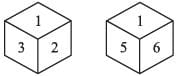Detailed Solution for Olympiad Test: Cubes and Dice - Question 7

From figure (i) & (ii) 2, 3, 5, 6 lie adjacent to 1. 4 lies opposite to 1.

Olympiad Test: Cubes and Dice - Question 8

In the given figure, when 4 is at the bottom, what number will be on the top?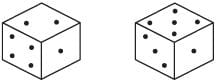Detailed Solution for Olympiad Test: Cubes and Dice - Question 8

When 4 is at the bottom then 3 will be on the top.

Olympiad Test: Cubes and Dice - Question 9

Two positions of a parallelepiped are given below. When the number 3 will be on the top side then which number will be at the bottom?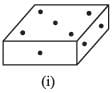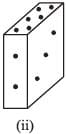Detailed Solution for Olympiad Test: Cubes and Dice - Question 9

If 3 will be on the top, then 5 will come at bottom.

Olympiad Test: Cubes and Dice - Question 10

Three different positions X, Y, Z of a dice are as shown below.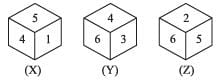Which number lies opposite to 6?

Detailed Solution for Olympiad Test: Cubes and Dice - Question 10

From positions X and Y we conclude that 1, 5, 6 and 3 lie adjacent to 4. Therefore, 2 must lie opposite 4. From positions Y and Z we conclude that 4, 3, 2 and 5 lie adjacent to 6. Therefore, 1 must lie opposite 6.

Olympiad Test: Cubes and Dice - Question 11

In the given figure, which number lies at the bottom face in position B?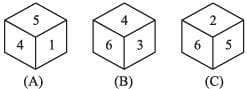Detailed Solution for Olympiad Test: Cubes and Dice - Question 11

From positions A and B we conclude that 1, 5, 6 and 3 lie adjacent to 4. Therefore, 2 must lie opposite 4. From positions A and B we conclude that 4, 3, 2 and 5 lie adjacent to 6. Therefore, 1 must lie opposite 6. Thus, 2 lies opposite 4, 1 lies opposite 6 and consequently 5 lies opposite 3.
2 lies at the bottom face in position B.

Olympiad Test: Cubes and Dice - Question 12

In the following figure, which symbol is opposite to the arrow.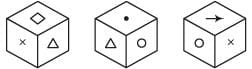Detailed Solution for Olympiad Test: Cubes and Dice - Question 12

(B) 'From Figs (i) and (ii) it is clear that triangle appears opposite arrow'.

Olympiad Test: Cubes and Dice - Question 13

Two positions of a block are given below. When 1 is at the top, which number will be at the bottom?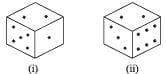Detailed Solution for Olympiad Test: Cubes and Dice - Question 13

2 is common to the two positions of the dice. If the dice in figure (i) is rotated so that 2 remains on the top face and the numbers 3 & 6 move to the face hidden behind 5 & 1 respectively. 5 lies opposite to 3 and 6 lies opposite to 1.

Olympiad Test: Cubes and Dice - Question 14

In the given figure, when 2 is at the bottom, what number will be at the top?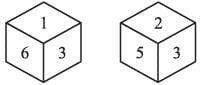Detailed Solution for Olympiad Test: Cubes and Dice - Question 14

When 2 is at the bottom 1 will be at the top.

Olympiad Test: Cubes and Dice - Question 15

Cubes of same size are arranged in the form of cube on a table then a column of five cubes is removed from each of the four cubes is removed from each of the four corners. All the exposed faces of rest of the cube are coloured yellow. Then how many small cubes are there in the solid after the removal of the column?

Detailed Solution for Olympiad Test: Cubes and Dice - Question 15

Required no. of cubes = 125 – (4 × 5)
= 125 – 20 = 105

## Science Olympiad Class 9

17 videos|6 docs|55 tests
 Use Code STAYHOME200 and get INR 200 additional OFF Use Coupon Code
Information about Olympiad Test: Cubes and Dice Page
In this test you can find the Exam questions for Olympiad Test: Cubes and Dice solved & explained in the simplest way possible. Besides giving Questions and answers for Olympiad Test: Cubes and Dice, EduRev gives you an ample number of Online tests for practice

## Science Olympiad Class 9

17 videos|6 docs|55 tests